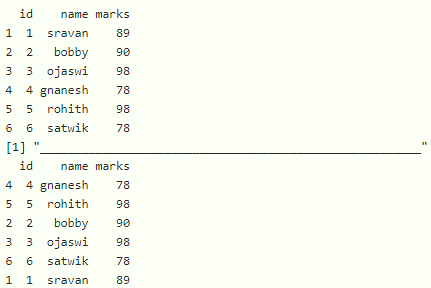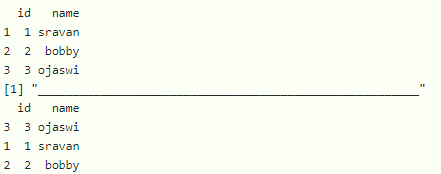# How to shuffle a dataframe in R by rows

• Last Updated : 14 Sep, 2021

In this article, we will discuss how to shuffle a dataframe by rows in the R programming language.

Shuffling means reordering or rearranging the data. We can shuffle the rows in the dataframe by using sample() function. By providing indexing to the dataframe the required task can be easily achieved.

Syntax:

dataframe[sample(1:nrow(dataframe)), ]

Where.

• dataframe is the input dataframe
• sample() function is used to shuffle the rows that takes a parameter with a function called nrow() with a slice operator to get all rows shuffled.
• nrow() is sued to get all rows by taking the input parameter as a dataframe

Example: R program to create a dataframe with 3 columns and 6 rows and shuffle the dataframe by rows

## R

 `# create a dataframe of students with id,name and marks``data=``data.frame``(id=``c``(1,2,3,4,5,6),``                ``name=``c``(``"sravan"``,``"bobby"``,``"ojaswi"``,``"gnanesh"``,``                       ``"rohith"``,``"satwik"``),``                ``marks=``c``(89,90,98,78,98,78))`` ` `# display dataframe``print``(data)``print``(``"_______________________________________________________"``)`` ` `# shuffle the dataframe by rows``shuffled_data= data[``sample``(1:``nrow``(data)), ]`` ` `# display``print``(shuffled_data)`

Output:Example: R program to create a student dataframe with 2 columns and 3 rows

## R

 `# create a dataframe of students with id,name``data = ``data.frame``(id=``c``(1, 2, 3), name=``c``(``  ``"sravan"``, ``"bobby"``, ``"ojaswi"``))`` ` `# display dataframe``print``(data)``print``(``"_______________________________________________________"``)`` ` `# shuffle the dataframe by rows``shuffled_data = data[``sample``(1:``nrow``(data)), ]`` ` `# display``print``(shuffled_data)`

Output:My Personal Notes arrow_drop_up